## //----------------------------// ArtMatic 1 in 1 out components //----------------------------//

Set Constant
Math tools #
Ax+B
Offset (x+A)
A - x
A/x
Abs x
Smooth Abs
A - Abs(x)
Mirror
Gaussian
Plateau
x squared
x power A
A power x
C Log (Cx+B)
Envelopes #
Power slope
A(1-exp(-x))
A/(xx+B-1)
Split & stretch
Sin x
Power sin #
Tile wave
Modulo
Saw waves #
U wave
x(Sin x)
Sin(Bx)/sin(x)
Sines cluster #
Tech Waves #
x + A sin Bx
Quantize
Smooth Steps
Variable Steps #
Sphere
Cubic Clip
Clip
Floor
Smooth Clamps #
Contrast
Semitones Seq
Grains
Discrete Random
Linear Random
Random
Periodic Random
Multi Perlin noise
Fractal noise
Randomize
Derivative
2D Derivative
Slope #
Compare
Iterations
Memory Logic#
Memory Maths
RGB Alpha Pack
11 Compiled tree

## Introduction

11 components are scalar (single output) functions. Most of them act as filters that modulate or remap the incoming values. They also provide basic building blocks for implementing your own algorithms and formulas. In many cases, the component's name is the calculation performed by the component. An essential in-depth discussion of ArtMatic structures Trees and components is found in ArtMatic Designer References and in Building trees.
In the discussion below, x signifies the tile's input value. It is often useful to insert 11 components between other components (and at the end of the tree) in order to adjust individual value 'streams'.

##11 Set Constant

parameters :
Algorithm dependent

discussion :
This component returns a constant value set by parameters regardless of the input value. The options popup provides three interpretations of parameter A: direct value, 1/value, angle in degree. When direct value is selected, the output value is the setting of parameter A. When the 1/value option is used, the output is 1 divided by the parameter A value. When the angle in degrees option is selected, parameter A is treated as a value in degrees, and the output value is the equivalent value expressed in radians.

algorithms :

##11 Math tools #

parameters :
No parameters.

discussion :
This is an advanced level component for implementing mathematical equations or optimizing the performance of a complex structure tree. This component performs a mathematical operation selected with Parameter A on the input. Parameter A should not be animated since it is a selector that determines what operation this component performs. When you move the parameter slider, the Tool Tips region will display the operation that corresponds to the slider value. Some of these (like sin) have equivalent components with more parameters. Functions that can be used as limiting envelopes use parameter B for scaling. Using parameter B essentially gives control over the envelope size. This functionality is useful when you want to limit the output of another component by multiplying the results. This parameter (B) applies to gauss: exp-x*x, clamp unity, cubic unity, and cubic unity in x-. In some cases, there are other 11 components that implement version of this function. When that is the case the this component's version (Math Tools #) will be fastest as it is optimized for speed. For instance, if you need a superfast sin(x) transform, use this one. The normal sin(x) has more parameters, but that flexibility comes at the cost of computation speed. The available functions (available either from the Algorithm popup or parameter A) are:

algorithms :
• x*x :
x-squared.
• sqrt(x) :
Square root of x. If x<0, the output is 0
• log(x) :
Natural log of x. Infinity when x<=0
• exp(x) :
e^x. Remember exp is the inverse of log.
• gauss : exp(-x*x) :
Gaussian curve

• x/Pi :
Divides input by Pi. Useful for music/sound application to tune unity-based functions to semitones pitch base. Far example "unit saw " (or saw wave with freq 1) can be tuned to same frequency as the sine wave just by adding the x/Pi above.
• x*Pi :
Multiplies input by Pi.

• sin(x) :
Simple sine function.
• cos(x) :
Simple cosine function.
• tan(x):
Arctangent.
• acos(x) :
Inverse of cosine function : ArcCos(x). The input values are 'tiled' to the range -1 to 1.
• asin(x) :
Inverse of sine function. The input values are 'tiled' to the range -1 to 1.
• atan(x) :
Returns the Inverse of tan(x) : ArcTangent(x).
• hyperbolic tan :
Returns the Hyperbolic Tangent(x) of input x.

• zero floor (x+) :
x is limited to values from 0 through infinity. If x<0, the output is 0.
• smooth zero floor (x+) :
x is limited to values from 0 through infinity. using a smooth max (x,0).
• smooth zero roof (x-) :
x is limited to values from - infinity to 0 using a smooth min (x,0).
• clamp unity :
clamp(x,0,1) Make sure output stays in 0 -1 range.
• cubic unity :
Cubic spline of x from 0 - 1 using (x*(x*(3-x-x))).
• cubic unity in (x-) :
Similar to the cubic unity algorithm (a cubic envelope limiting values to the range 0-1) but acting on on the negative part of x-axis.
• unit mod (fract(x)):
Returns the fractional part of the input which is equivalent to modulus1. Modulus has a sharp discontinuity when signal jumps from max to min.
Soundwise it is a saw tooth signal that has a rich frequency content.
• unit saw :
Unbalanced unit (from 0 to 1)triangle wave.
• unit smooth saw :
Unbalanced unit (from 0 to 1) triangle wave with smoothed edges.
• balanced unit mod :
Balanced modulus from -1 to 1. When the input is 0, the output is 0. This is a classic ramp wave.
• balanced unit saw :
Balanced unit (from -1 to 1) triangle wave.
• balanced smooth saw :
Balanced unit (from -1 to 1) saw wave with smoothed edges.

• N-abs(x) :
Returns a triangle envelope where peak N is located at input x =zero and sides goes linearly towards minus infinity.
• roofed N-abs(x) :
Returns a triangle envelope whose peak is clamped to 1. When N is above 1 it will create a trapezoidal profile.
• dent : min(x abs(x)-N) :
Returns the minimum of x and a inverse triangle envelope (peak pointing down). When N is at zero the 'dent" disappears and x is left unchanged.

• iteration break (|x|>32) :
This algorithm aborts iterations within a recursive compiled tree when the input value is larger than 32. This can be used to optimize certain fractals by aborting meaningless (and time-consuming) iterations.

Note : Use the 'Utility function plot" tree to see the plot of each function.

##11 Ax+B

parameters :
A : Scaling (-20. : 20.)
B : Offset (-32. : 32.)

discussion :
This linear scaling component multiplies the input with parameter A then adds an offset (parameter B). It is nice for adjusting the contrast and level of a system. When the input is packed it will process the RGB channels and return a packed vector of same dimension.

Parameter options:

• Direct value
• 1/value
• Angle in degrees
• Semitones
• Value*10
• Value/10

• When 'direct value' is selected, the output value is simply the setting of parameter A.
When the '1/value' parameter option is used, the output is 1 divided by the parameter A value.
When 'angle in degrees' is chosen, parameter A is treated as a value in degrees and the output value is equivalent value expressed in radians. The angle in degrees option is convenient for multiplying by Pi (180 degrees) or Pi/n. The range of 'Offset' parameter goes then from -180 to 180.
The option 'semitones' is useful for sound synthesis. It sets A to exp(A*log(2)/12) which is the ratio of a temperated scale semitone A. By scaling the input of a wave tuned to default you can transpose the pitch by semitones with zero being the fundamental, 2 one tone above, -3 a minor third below. A needs to be an integer to obtain a real semitone frequency. Of course the tuning will be relative to the fundamental frequency, so make sure it is set to a known value (Preferences dialog gives the tuning of a sine at frequency 1 in semitones where A0 is 441 hertz).

##11 Offset (x+A)

parameters :
A : Offset (-64. : 64.)

discussion :
Adds a value supplied by the Offset parameter to the incoming value. When the input is packed it will process the RGB channels and return a packed vector of same dimension.
This component has various parameter options to adjust the range or nature of the offset: direct value, 1/value, angle in degrees, value *10 and value *20, value /10 and value /20. The last options are handy for larger or smaller displacements. When 'angle in degrees' is chosen the range of 'Offset' parameter goes from -180 to 180, which is -Π to Π.

##11 Reverse : A - x

parameters :
A : Level (0. : 20.)

discussion :
This component revert the direction of the input values. When A is zero, this component just returns the negative of the input.
There are various parameter options to adjust the range or nature of parameter A:  direct value, 1/value, angle in degrees, value *10 and value *20, value /10 and value /20.
For example to obtain exactly Π-x you will set the option to "angle in degrees" and use 180 for parameter A.

##11 Inverse : A/x

parameters :
A : Amplitude (-10. : 10.)

discussion :
A/x returns the inversion of the input scaled by A. Note that with x close to zero the output will tend to infinity.

algorithms :
• inverse 1/x :
inverse 1/x.: returns A/x where A is the value of parameter slider A and x is the input value.
• contours : 1/abs(x) :
returns A/abs(x) instead of A/x. This algorithm is great for neon effects as the light will slowly vanish when x increases and is symmetrical around zero.

##11 Abs x

parameters :
A : Offset (-32. : 32.)

discussion :
Returns the absolute value of the input (i.e. it strips the minus sign from the input) plus the value of parameter A. When applied to a space coordinate, Abs X will create a mirror symmetry. The various options allows to orient the mirroring and change the range of the offsets parameters.
Formula: |x| + A or A -|x| depending on mirror orientation.

##11 Smooth Abs

parameters :
A : Smoothness % (1/64 : 8.)
B : Offset (-32 : 32)
C : Phase (-10 : 10)

discussion :
This is a modified version of the absolute value function which is smoothed near the origin. 'Smoothness' controls the degree of smoothing while 'Offset' offsets the result after the absolute value. The 'Phase' parameter, introduced in ArtMatic Engine 8.0, offsets the input prior the absolute value. This is often handy to adjust the position of the mirror if the input is not balanced. The various options allows to orient the mirroring and change the range of the offsets parameters.
Formula: smoothAbs(x+C) + A or A -smoothAbs(x+C) depending on mirror orientation.

##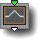11 A - Abs(x)

parameters :
A : Size (0.06 : 4.)
B : Offset (-8. : 8.)
C : Scale (1. : 16.)

discussion :
Similar to mirror below A- Abs X floor the result to zero so the output is positive only.

##11 Mirror

parameters :
A : Scaling (0. : 4.)
B : Offset (-32. : 32.)
C : Smoothing % (0. : 4.)

discussion :
A symmetry component that creates a mirror of the input values. The Offset parameter moves the mirror axis. Parameter A scales the output value and should be kept at 1 for a true mirroring. Formula: if (x>B) then y = 2 * B - x

##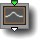11 Gaussian

parameters :
A : Scale (0. : 128.)
B : Phase (-16. : 16.)
C : Amplitude (0. : 8.)

discussion :
The Gaussian tile returns a bell curve. The output of a Gaussian function at 0 is 1 and approaches 0 when A(x+B) is greater than 2. To increase the width of the bell curve, decrease parameter A. The curve is symmetrical around x=0. Formula: C exp(-X^2) where X is A(x+B)

##11 Plateau

parameters :
A : Slope (0.03 : 8.)
B : Size (-4. : 20.)
C : Amplitude (-8. : 8.)

discussion :
Plateau is a gaussian-type filter with an extended plateau on either side of 0. It is useful for delineating symmetrical shapes and creating edge effects. See the file Flying Rectangles to see how plateau can be used to provide rounded corners. Slope determines the sharpness of the plateau edge. Size determines the plateau width. 'Amplitude' is a multiplier that sets the plateau height.

##11 x squared

parameters :
A : Amount (0. : 1.)
B : Scale (1. : 32.)

discussion :
Another mathematical primitive that returns x-squared. When the amount parameter is set to the maximum, the output is the input-value squared for input values above 0 and 0 for input values below 0. Otherwise, amount blends the unfiltered input value with the squared version. The 'Scale' parameter is applied to the input value before it is squared. Formula: mix(x , x>0 ? Bx^2 : 0, amount)

##11 x power A

parameters :
A : Power (1/8 : 64.)
B : Scaling (1/256 : 32.)

discussion :
This component provides non-linear remapping of values. With the power parameter set to the minimum, you can get useful discontinuities such as well-defined islands and borders (depending on the input). The range of the output in respect to 'Power' is from the eighth root of x to the 64th power of x. If the incoming value is negative, the absolute value is taken before applying the function. High power are handy for metallic shaders.

algorithms :
• Scaled power (x^A)/(B^A):
(x^A)/(B^A)": Parameter B is applied as an additional scaling factor.
• Power+ x^A:
Straight power.

##11 A power x

parameters :
A : A (0.03 : 4.)
B : Amplitude (0.03 : 2.)

discussion :
Another basic mathematical building block : it returns the x power of parameter A. Formula: B * (A^x).

##11 C Log (Cx+B)

parameters :
A : Amount (0. : 1.)
B : Offset B (0. : 2.72)
C : Scale C (0.06 : 4.)

discussion :
This filter applies the natural log to the input. It is good for decreasing the output range of functions that go to infinity. The amount parameter determines the steepness of the output curve.

##11 Envelopes

parameters :
A : Peak point % (0. : 1.)
B : Decay time % (0. : 4.)
C : Amplitude (0. : 8.)

discussion :
This component creates an envelope with a given attack and decay with various shapes. Values outside of the envelope range are mapped to zero. Thus Envelopes outputs a signal that always starts and ends at zero. This component can serve as a nice contrast or surface contour control. It can also serve as an envelope filter for sound design when multiplying its output with the sound waveform. The 'Amplitude' essentially sets the height of the saw envelope. As an envelope is often used to shape another signal 'Amplitude' is often left at 1. the Slope or 'Decay time' 'parameter sets the decay length. 'Peak point' or offset sets the attack time. The Right Slope parameter (A) may have a value less than 0. A negative slope provides a gradual attack and a rapid decay rather than the a quick attack and slow decay. In general the algorithms maps the interval 0 : 1 to the envelope and sets elsewhere to 0. All curves are continuous except saw which is linear. Note the Amplitude scales also the frequency so you can have the envelope works on the range 0-3 if you set it to 3. Some decay time (oriented saw and impulse) can be longer than the 0-1 window.

algorithms :
• Oriented saw:
A triangle (linear) wave with a faster attack than decay.
• Impulse:
Now the default. parameters : peak point, decay time, amplitude.
• Asym power:
Parameters : power left, power right, amplitude.
• Power decay:
Parameters : peak point, power right, amplitude.
• Parabola:
Symmetrical. parameters : power , amplitude.
• Gate :
Sharp slope in and out envelope. parameters : slope , decay point.Impulse envelope with a peak at 0.1 and a 0.45 decay time.

##11 Power slope

parameters :
A : Size (0.12 : 8.)
B : Phase (-32. : 32.)
C : Power (0.12 : 16.)
D : Amplitude (-8. : 8.)

discussion :
An exponential curve with a step function-like effect except that it is continuous with rounded edges near the 'step". This function, when used at the final stage of a system, tends to make the image very high contrast -- black and white rather than shades of gray, for example.

##11 A(1-exp(-x))

parameters :
A : Amplitude (0.12 : 8.)

discussion :
This component filters the input with an inverted Gaussian curve. It provides a convenient way to smoothly clip values that approach infinity. It is a little like log, but has a steeper slope and sharper cutoff.

##11 A/(xx+B-1)

parameters :
A : Amplitude (0 : 2.)
B : Offset (1.0001 : 2.)

discussion :
This component, changed in Armatic engine 8.06, is similar to Gaussian in the sense the result is symmetric and its maximum occurs when x is zero. It has sharp spike around origin and the fallout is a good approximation of physical system that fades according to the inverse square power of the distance. Thus it is often applied to a 21 distance function to get lights effects.
Formula: A/(x^2+B-1).

##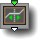11 Split & stretch

parameters :
A : Amount (-32. : 32.)
B : Offset (-16. : 16.)
C : Smooth (0. : 1.)

discussion :
This handy component divides the input about 0 and pushes apart the positive and negative values. It is very useful for modeling shapes. For example, insert an instance of this component before both the left and right inputs of a circle, and the result is a rounded rectangle.

##11 Sin x

parameters :
A : Amplitude (-16. : 16.)
B : Phase (-12.57 : 12.57)
C : Frequency (-16. : 16.)

discussion :
This component returns the sine of the input which renders its output periodic (cyclic). It is the basic primitive for pitched sounds and any periodic phenomenon. It can be used for sound creation, wood-type textures, zebra patterns and much much more.

##11 Power sin

parameters :
A : Frequency (-8. : 8.)
B : Phase (-12.57 : 12.57)
C : Power (0. : 16.)
D : Amplitude (0. : 4.)

discussion :
This periodic component is useful for creating banding effects and for adding harmonics when in Sound mode. It is equivalent to passing the sin output through a power filter. 'Amplitude' scales the output amplitude while 'Frequency' scales the input.
Formula: (sin (x*A+B))^C.

algorithms :
• power sin (square):
At power lower than 1 the signal converges toward a pure square wave (C=0).
• saw power sin (saw)
At power lower than 1 the signal converges toward a pure saw tooth wave (C=0).

##11 Tile wave

parameters :
A : Amplitude (0. : 16.)
B : Phase (-12.57 : 12.57)
C : Frequency (-16. : 16.)

discussion :
A non-symmetrical sawtooth wave. Very handy for creating repetitive patterns and for creating sound waves. With default parameter values, it repeats the interval bounded by -1 and 1 which keeps the output centered about 0 (essential for musical applications). If you place a Tile Wave component before any other 1D filter, the filter will become periodic (though not necessarily continuous since the ramp drops immediately from its maximum value to its minimum value). For example, if you need a repeating figure of gaussian grains, put a Tile Wave before the Gaussian tile. Tile Wave is similar to modulo but without having the amplitude proportional to frequency.

Sound tip: Multiply the result with a sine wave and you get rich sounds.

##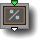11 Modulo

parameters :
A : Period (0. : 100.)
B : Phase (-100. : 100.)
C : Amplitude (0. : 16.)

discussion :
Modulo returns the modulo/remainder function of input. Applied on x it will create a saw tooth signal that repeats the 0-A interval.

It is very useful when you need to repeat a pattern or create a periodic function. The balanced form can be used for DF modeling when keeping amplitude at 1. Frequency determines the spacing of the created 'ramps" (by setting the divisor used to generate the modulo). Amplitude is applied at the output stage.

algorithms :
• Infinite modulo balanced:
Balanced infinite modulo with output range (- 'Amplitude', + 'Amplitude').
• Infinite modulo +:
Positive only infinite modulo with output range (0, + 'Amplitude').
• Repeats N :
Limits the modulo function to a particular number of repetitions (rather than go on infinitely as it does with the default Infinite Modulo algorithm). The fourth parameter, Number of Repeats, is active for this algorithm. This algorithm is convenient for creating finite repetitions in X or Y by inserting it on the appropriate axis. 0 is in the middle of each repetition series.

DFRM NOTE: When using Repeats N with DFRM, leave the Amplitude at 1 in order to maintain the distance field's integrity. Parameter options: Direct values (default), 1/scale, scale * 10, offset: value*10, offset: value*20, offset & scale * 10 In the parameter option names, Scale refers to parameter A's value and Offset to parameter B's value. For example, offset & scale * 10, will set both parameter A and parameter B to 10 times the sliders' nominal value whereas Scale * 10 treats parameter A as 10 times its nominal value but interprets parameter B normally.

##11 Saw waves #

parameters :
A : Frequency (-32. : 32.)
B : Phase (-100. : 100.)
C : Amplitude (-16. : 16.)
D : Smoothness % (0. : 1.)

discussion :
This periodic component returns a triangle wave function similar to a sine wave but with sharper edges. Saw wave is often used in sound synthesis for the richness of its harmonic content. When used on a space coordinate input, the resulting space will feature a periodic mirroring symmetry on the corresponding axis.

The repeats and Folding modes will limit the mirroring of saw to a discreet numbers of mirroring, thus a finite number of repetitions (set by parameter D) are performed. The Repeats and Folding are useful for design, architecture and kaleidoscopic 2D or 3D fractals.

For DF modeling N Fold asymmetry is particularly useful to repeat a zero centered object.With Repeats (red curve) the result is symmetrical around zero and zero x maps to +-C
With Foldings (green curve) the space around zero is left unmodified zero x maps to 0

algorithms :
• Infinite saw balanced:
Returns a saw wave in -Amplitude + Amplitude range.
• Infinite saw +:
Returns a saw wave in 0 - Amplitude range.
• Saw displace:
Add the saw wave to the input.
• Repeats N, Hard edges:
Does a limited amount of saw repetitions given by parameter D when in this mode.
• Repeats N, Smooth edges:
Same as before but with smooth edges.
• N Fold asym:
N repeats with zero untouched. Parts before and after folding are left unchanged.
• N Fold smooth asym
Same as before but with smooth edges.

Parameter options:

• "Default : direct values"
Frequency will be the given value A
• "Use Pi based period"
useful for sound design : frequency of 1 will be Pi, thus saw will be cycling like a sine
• "Use 1/scale"
practical for very low frequencies
• "Use scale * 10"
• "Use 1/scale * 10"
practical for very very low frequencies

##11 U wave

parameters :
A : Frequency (0. : 8.)
B : Phase (-12.57 : 12.57)
C : Amplitude (-4. : 4.)

discussion :
This component is a useful u-shaped periodic wave based on the absolute value of a sine function.

##11 x(Sin x)

parameters :
A : Frequency (0. : 16.)
B : Phase (-6.28 : 6.28)
C : Amplitude (0. : 4.)

discussion :
This component is a variation of the sine function where the amplitude of the sine wave is scaled by the input x. If the input is itself periodic, x sin(x) can be used to create interesting modulated sounds.

Formula: x + A sin(Bx)

##11 Sin(Bx)/sin(x)

parameters :
A : Frequency (0. : 8.)
B : Harmonics (0 : 16)
C : Amplitude (0. : 2.)

discussion :
This component is great for creating harmonics for structures designed for sound synthesis (when using the Direct Drones synthesis method). The component equation is Sin(Bx)/sin(x). Amplitude determines the output amplitude. Frequency influences the harmonic spacing. Harmonics determines the number of harmonics.

##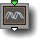11 Sines cluster

parameters :
A : Frequency (-16. : 16.)
B : Spread % (0. : 1.)
C : Amplitude (0. : 2.)

discussion :
This series generates various sounds based on the integration of 24 sines waves. Nice fuzzy and voicy sounds can be obtained with sines clusters.

algorithms :
• Fuzzy Harmonics:
Parameters : freq, spread, amplitude. Spread controls how much individual sines are detuned from the center freq.
• Gauss Randomized :
Parameters : frequency, spread, amplitude. Randomization follows a gaussian distribution.
• Harmonics subset gaussian:
Parameters : frequency, spread, amplitude, harmonic offset. Integrates part of the harmonic series starting at harmonic offset with a Gaussian amplitude distribution.
• Harmonics subset 1/f,
Harmonics*2 subset 1/f,
Harmonics*3 subset 1/f:

Harmonics subset 1/f Integrates part of the harmonic series starting at harmonic offset with a 1/f amplitude distribution (the higher the lower). The *2 and *3 is the spacing between harmonics. For example if H offset is 2 and H*3 is used we will get (2, 5, 8, 11… etc) for 24 sines.
Parameters : freq, amplitude, harmonic offset.

##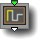11 Tech Waves

parameters :
Algorithm slider : (0 : 26)
B : Amplitude (0. : 16.)
C : Frequency (0. : 32.)

discussion :
Tech waves is primarily used for creating audio. Parameter A selects between different waveforms with various level of smoothing. The Tool Tip displays the waveform name as you move the parameter slider. The waveforms range from a standard square wave to random waves great for creating interesting noise. There are 3 flavors of smoothness for each oscillator type which is essentially high frequency filtering.

algorithms :
• Square Pulse :
• T Pulse :
• Saw Pulse :
• Sparse Pulse A:
• Sparse Pulse B:
• Alternate :
• Cyclic Random 8 :
• Cyclic Random 16 :
• Random :
As they are hard to describe we suggest to use The 1D function plot utility and set the top function to one of them.

##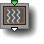11 x + A sin Bx

parameters :
A : Amplitude (0. : 2.)
B : Frequency (-16. : 16.)

discussion :
This component modulates (or displaces) the input with a sine function rather than simply returning the sine function. Formula: x+ A sin(Bx)

##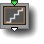11 Quantize

parameters :
A : Frequency (0.12 : 16.)
B : Amount (0. : 1.)
C : Smoothness % (0. : 1.)

discussion :
Quantize the input into discrete steps. It turns a gradual gradient, for instance, into a series of color bars as in the illustration below. Use this function to create discontinuous, step-like output. It is great for creating pixelization and color-reduction effects. Quantize is not continuous and not derivable and thus is not advised for ArtMatic Voyager or sound design.

Frequency determines the spacing of the quantization steps. The Amplitude determines the strength of the quantization. (With a value of 0, there is no quantization; with a maximum value, true quantization is performed.)The image on the left is the result of applying the Quantize component to a gradual ramp (provided by the Ax+By+C component). The image on the right is the un-quantized image.

##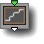11 Smooth Steps

parameters :
A : Amplitude (0. : 1.)
B : Randomness (0. : 1.)
C : Frequency (0.06 : 8.)

discussion :
This component is a quantization function that has smooth transitions between the steps (unlike Quantize which has discontinuous steps). This component is especially useful for designing systems for use with ArtMatic Voyager which dislikes the discontinuities introduced by the Quantize component.

When the Randomness parameter is non-zero, the steps are spaced irregularly, yielding a natural look. This component is often used to create ArtMatic Voyager terrains to achieve eroded terraces such as those shown in the canyon example shown below.##11 Variable Steps

parameters :
A : Random Steps soft # (0 : 7)
B : Amplitude (0. : 16.)
C : Frequency (0. : 32.)

discussion :
Variable Steps is a randomized quantizing (step) function that combines two step sizes with varying degrees of smoothness. Parameter A is used to select the quantizing algorithm. This component is especially useful for creating terraced landscapes in ArtMatic Voyager.

##11 Sphere

parameters :
A : Radius (0.1 : 10)

##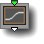11 Cubic Clip

parameters :
A : Amplitude (0. : 16.)
B : Offset (-8. : 8.)
C : Slope (-50. : 50.)

discussion :
Cubic clip clamps the input between 0 and 1 using a cubic spline (x*x(3-2*x)) to maintain continuity at the clipping boundaries. 'Offset' is added to the input before applying the cubic filter while 'Slope' parameter scales the input value prior the clamping. 'Amplitude' scales the result so that the final output range will be positive only and between 0 and amplitude A.

##11 Clip

parameters :
A : Contrast (0.12 : 8.)
B : Offset (-8. : 8.)
C : Cutoff (0.25 : 16.)

discussion :
This filter clips the input when it passes outside of the range from 0 to the value defined by the CutOff parameter. 'Offset' is added to the input before applying the Cutoff. Resulting range will be positive only and between 0 and 'Cutoff'.

##11 Floor

parameters :
A : Floor level (-100. : 0.)
B : Roof level (0. : 100.)

discussion :
Constrain the input to values between the Floor Level and the Roof Level. Any value below the Floor Level is assigned the floor level. Any value above the roof level is assigned the roof level.

##11 Smooth Clamps #

parameters :
A : Floor level (-100. : 32.)
B : Roof level (-32. : 100.)
C : Smoothness % (0. : 4.)

discussion :
"Smooth Clamps" provides several clamping functions where the 'floor level' and 'roof level' parameters set the lower and upper limits. These functions are great for limiting any kind of inputs to a specific range.

algorithms :

Smooth Floor->clamp applied to a fractal surface creates convincing plateaus in ArtMatic Voyager terrains.##11 Contrast

parameters :
A : Contrast amount (-3. : 1.)
B : Offset (-4. : 4.)

discussion :
A simple, but very useful, contrast filter. When Amount (parameter A), is set to the maximum, there are only two possible output values (i.e. if this is the last component in a system, the result will be a two-tone image). The Offset parameter controls where the contrast divide is made. When used to control picture luminance values, offset effectively adjusts the overall threshold level. See Concentric dither BW for an example.

##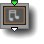11 Semitones Seq

parameters :
A : Loops (2. : 32.)
B : Phase (0. : 64.)
C : Amplitude (0. : 8.)

discussion :
This component is used to create random note sequences in Sound mode. Generally, this component is connected to one of the time outputs of the Global Input Matrix (as shown below) which causes the notes to vary over time. The output values are limited to semitone values when used in a well-behaved sequence. See the examples provided with ArtMatic.
Example files: QT Mod Seq., Master LFO Test series of examples.
Parameter details
'Loops': The number of loops (notes) that will be created in a given time frame. Larger values result in phrases with more notes.
'Phase': An offset within the random sequence. By changing the phase, you can create more complex musical phrases since the sequence will not simply repeat over and over.
'Amplitude': Determines the pitch range of the notes from which the sequence will be generated.##11 Grains

parameters :
A : Amplitude (-16. : 16.)
B : Size (0. : 1.)
C : Frequency (0. : 8.)
D : Phase (-32. : 32.)

discussion :
Grains is a non-periodic function that randomly distributes small gaussian-shaped 'grains". It is useful for both graphics and sounds. For graphics applications, use it when you need a series of lines that is not densely spaced. For sound applications, use it to create narrow-band noise that sounds more brassy than white noise or fractal noise.

##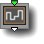11 Discrete Random

parameters :
A : Amplitude (-8. : 8.)
B : Phase (-32. : 32.)
C : Frequency (0. : 16.)

discussion :
This component returns a random step function of input x. It is not continuous and not derivable and thus is not advised for ArtMatic Voyager or sound design.

##11 Linear Random

parameters :
A : Amplitude (-8. : 8.)
B : Phase (-32. : 32.)
C : Frequency (0. : 16.)

discussion :
A simple discontinuous random function with linear interpolation. Use this component for creating high order harmonics for sound synthesis or when you need sharp edges.

##11 Random

parameters :
A : Amplitude (-8. : 8.)
B : Phase (-32. : 32.)
C : Frequency (0. : 16.)

discussion :
This component generates a band-limited and continuous random function. Formula : A Random(B(X+C)). Random is simpler and faster than a Perlin Noise.

##11 Periodic Random

parameters :
A : Amplitude (-16. : 16.)
B : Phase (-32. : 32.)
C : Frequency (0. : 16.)

discussion :
A periodic (repeating) random function particularly useful for sound applications. It is band-limited and richer than a pure sine wave. The phase parameter adjusts the contour of the random output.

##11 Multi Perlin noise

parameters :
A : Amplitude (-8. : 8.)
B : Octaves (1. : 128.)
C : Frequency (0. : 32.)
D : Roughness (0.12 : 0.75)

discussion :
This component sums a series of perlin noise functions with the frequency doubling for each function in the series. The octaves parameter determines the highest frequency generated. When the octaves parameter is at its maximum (128 which is is 27) there are seven 'octaves" of noise. Multi Perlin Noise is a band-limited noise function very useful for sound synthesis as the upper frequency limit can be controlled by the octaves parameter.

##11 Fractal noise

parameters :
A : Amplitude (-8. : 8.)
B : Phase (-32. : 32.)
C : Frequency (-16. : 16.)
D : Roughness (0.30 : 0.60)

discussion :
A 1D version of the fractal noise algorithm. See the descriptions of the higher-dimensional fractal noise components for more information. Besides many graphical applications, Fractal noise can also be used as a sound source to produce 'pink noise" where the amplitude of specific frequencies follow the '1/f' rule. (Unlike 'white noise' which has all frequencies at the same amplitude.). Fractal noise produce a natural and pleasant un-pitched sound. Parameter D, Roughness, controls the fractal dimension. The default 'normal' value is 0.5.

##11 Randomize

parameters :
A : Amount (-8. : 8.)
B : Phase (-32. : 32.)
C : Frequency (0. : 16.)

discussion :
Randomize displaces input coordinates with a 1D continuous random noise by adding it to the input. 'Amount' controls the displacement amount while 'Frequency' and 'phase' sets the scale and offset of the 1D random noise. This differs from the Random function which generates truly random values.

##11 Derivative

parameters :
A : Amplitude (0. : 2.)
B : Offset (-2. : 4.)

discussion :
This component approximates the derivative of the function whose output feeds the derivative component. It is often used to emphasizes the 3D aspect of a system. Its output only makes sense when the input values are continuous (i..e. it does not work well with discontinuous & non-derivable functions such as Random Squares or discrete Random).

Note: This component will behave differently when it is in a compiled tree than when it is in the main tree since it uses information other than that which comes in through its input. When this component is used (almost always in the lower part of tree), ArtMatic traverses the tree for the information that it needs to derive the lighting information. As a result, this component can't be used inside of compiled trees since the tree as a whole is hidden from compiled trees. In short Derivative needs to 'see' the tiles above to evaluate the derivative properly.

##11 2D Derivative

parameters :
A : Light Direction dx (-2. : 2.)
B : Light Direction dy (-2. : 2.)
C : Offset (-4. : 4.)

discussion :
Related to the derivative, this function provides lighting and 3D texture effects by scanning the entire tree above it to compute its partial derivatives. Parameters A and B control the direction of the virtual light vector. When A is 1 and B zero the light comes from the left.When B is 1 and A zero the light comes from the top. This component actually looks at the entire tree structure and not just the incoming values. Note the 3D effect it has on a simple 2->1 Grid.

Note: This component will behave differently when it is in a compiled tree than when it is in the main tree since it uses information other than that which comes in through its input. When this component is used (almost always in the lower part of tree), ArtMatic traverses the tree for the information that it needs to derive the lighting information. As a result, this component can't be used inside of compiled trees since the tree as a whole is hidden from compiled trees.

Advanced note for the mathematically inclined: This component approximates the partial derivative along both the x and y axes of the system (dfx and dfy). The result is the dot product of parameters A and B with the approximated partial derivatives over x and y. This components is computationally-intensive as it will make the tree above render several times.

##11 Slope #

parameters :
A : Amplitude (0. : 8.)
B : Offset (-1. : 1.)

discussion :
This component provides a few algorithms options for calculating the slope of the terrain or 3D field that feeds it. The slope is linked to derivative and a value of zero means the surface is flat. As with the derivative component the 11 slope needs to 'see' the tiles above to evaluate the slope so you can't put it in a compiled tree to evaluate slope of functions located in the main tree.
The 2D Slope modes are meant to be used with 2D systems, while the 3D Slope is meant for volumetric 3D systems.
The mode 3D Slope can be used with 3D terrain to modulate terrain geometry according to the slope of one 3D function. When the Slope is used only for the shading phase when rendering in Voyager use the 13 derivative coming from voyager at no extra cost instead and get the slope by using (1-abs(dy)), dy being the y output of the derivative.

Options:

##11 Line Shaders

parameters :
A : Contrast % (0. : 100.)
B : Width (0. : 8.)

discussion :
11 line offers most of the shading option that the new 21 line offers plus some packed RGBA shaders so you can use the same component for color graphics. See the 21 Line discussion for the various algorithms. Unlike the 21 Line that rotates the coordinates to set the line slope, the 11 Line creates the line at the zero crossing of the input value surface. If you feed a 21 Interpolate using Rotate interpolator before the line you get exactly the same functionality as the 21 line. Connecting the 11 Line to x you will get a vertical line and an horizontal line to y.

The beauty of 11 Line is it will create a line for anything as soon as the input is balanced and crossed zero (+ and - so there IS a zero crossing). So if you feed the 11 line with a Disc profile you will get a circle. The Line will have a steady width when the input is DF compliant and not too distorted. For improving Line quality for contours of surfaces you can use the technique showed in Utility Contour Plot preset as shown in the example Libraries/Components demo/LineShader Surface Contour.

On top of the shaders used by the 21 Line 11 line has also the option to shade them as color half planes, treating the positive side of the line as a fill area for Packed RGBA fill + and the negative side with Packed RGBA fill -. It can also shade the line AND the fill(+) with the Packed RGBA line & fill ago.

lines Types:

##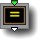11 Compare

parameters :
A : Value (-64. : 64.)
C : Output Value (1./8. : 64.)

discussion :
This component provides a way to compare an input value to a single value or value range. Where the input value matches the selection criterion, parameter C's value is passed out. Everywhere else 0 is passed out.

algorithms :
• Equal Exact value:
True if the input value exactly matches parameter A.
• Inside Range:
True if the input is inside the range bounded by parameter A and parameter A + parameter B.
• Outside Range:
True if the input is outside the range bounded by parameter A and parameter A + parameter B.
• Greater than:
True if the input is greater than parameter A.
• Less than
True if the input is less than parameter A.

##11 Iterations

parameters :
A : Multiplier (0. : 8.)
B : Iterations (0 : 500)
C : Start value (-4 : 4)

##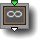11 infinity gate/mask

parameters :
A : Maximum (0. : 256.)
B : Minimum (-256. : 0.)
C : Invert mask (-1. : 1.)

discussion :
This function allows you to create or manage infinities. Infinity has some special characteristics: it is replaced by the
Depth cue color in RGB shaded systems) and infinities are treated as transparent in 'packed' mixers and most mathematical operators which provides implicit masking.

algorithms :
• Pass infinity:
When the input value is less than the minimum or greater than the maximum the value 'infinity' is sent out instead which makes those area transparent in most cases.
'Invert Mask' can invert the component's effect. When the parameter's value is greater than one, infinity is generated where the input value is between the min and the max parameters.

This is great for creating implicit masking and to optimize calculations in certain areas as most component will simply pass out infinities without computing their normal operations.
Infinities are mapped to parameter A value, thus discarding any infinities (Engine 8.07). In this mode 'Invert Mask' is disabled.

##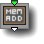11 Memory Add

parameters :
A : Start Value (-1. : 1.)
B : Sum Gain (0. : 2.)
C : Auto Gain % (0. : 1.)

discussion :
Memory Add simply adds the new value to the old value. It acts as an accumulator. The 'Start Value' parameter is the initial value which is added to the first iteration. Because the effect accumulates over the iterations, you may sometimes want to set the Start Value to a low value so that the system doesn't attain large/max values everywhere. 'Sum Gain' scales the output. Memory Add is basically a summation tool.
When 'Auto Gain' is set at 1 the weight will be calculated using the context iteration max to keep the sum independent of the iteration number. Lower values will blend with the 'Sum Gain' setting.
Infinities are discared and treated as transparent.
Recursion formula : memx= x+ memx with 'memx' being the accumulated output value.

##11 Memory Fractal Add

parameters :
A : Power slope (0.06 : 2.)
B : Sum gain (0. : 2.)

##11 Memory Logic#

parameters :
A : Smoothness % (0. : 16.)

discussion :
This component is only meaningful in iterative/recursive systems. It will accumulate input values according to a logic operation defined by the algorithm choice.

algorithms :

##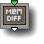11 Memory Maths

parameters :
A : Smoothness, Gain or Blend % (0. : 2.)

discussion :
This memory component accumulates scalar values using various mathematical functions provided by the following algorithms :

algorithms :

##11 RGB Alpha Pack

parameters :
A : Red Value % (0. : 1.)
B : Green Value % (0. : 1.)
C : Blue Value % (0. : 1.)

discussion :
This component either filters or creates a packed RGB-A stream depending on whether the input is a scalar (single) value or an RGBA stream. This is a convenient way to add a solid color to a DF object or to filter an existing stream's colors.Simple solid color for DFRM. If the input is a single scalar value, an RGB-A stream is created. The stream's RGB values are the values of parameters A, B and C and the alpha channel is the input value. Typically, this is used to create DFRM colored solid where the sliders 1, 2, and 3 determine the color, and the input provides the object (DF field). Using 11 RGB Alpha Pack is more efficient than using the 14 Set Constant component followed by a 4->1 Pack component.Packed Input. When the input is a packed RGBA stream, parameters A, B and C scale the input stream's RGB values and the alpha value is passed through unchanged. This is very handy for scaling a set of packed values.

## 11 Compiled Tree :

parameters :
A : Blend (0:1)
B : Recursions

discussion :
Compiled Trees are groups of tiles that can be used in place of single tiles as a kind of macro or subroutine. 11 Compiled Tree can be seen generally as a scalar valued 1D function unless the output is a packed vector in which case it can be a 3D or 4D vector valued 1D function. When packing 3D outputs you may use 11CT to define a 3D motion paths or a 3D parametric curve that can be rendered efficiently using the
3D Motion Path render component. With scalar outputs you can create complex filters that combines several 11 filters and treat them as a single tile that can be copied and pasted elsewhere or saved on disk using "Save compiled tree" from the Tree Edit menu.

usage :
Select a 11 tile and use "New compiled tree" to create a new CT from the selection (Tree Edit menu or type 'n' key). Parameter 'Blend' 'allows to mix between the input and the output and is set at 1 by default.
CTs can be used recursively if the option "Allow feedback" is set. In that case output is fed to the input at second iteration and the transform will be applied "i" times, i being set by the 'Recursions' parameter. 11 CT don't allows recursion with packed RGBA streams but the input or the output of the CT can be a packed vector. For example you can use a 11 CT to add all elements of a vector and return a scalar value.